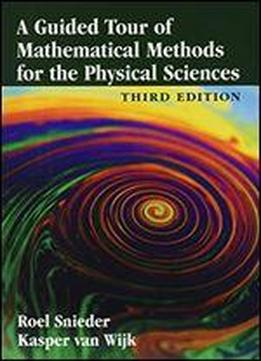# A Guided Tour Of Mathematical Methods For The Physical Sciences by Kasper van Wijk / 2015 / English / PDF

Mathematical methods are essential tools for all physical scientists. This book provides a comprehensive tour of the mathematical knowledge and techniques that are needed by students across the physical sciences. In contrast to more traditional textbooks, all the material is presented in the form of exercises. Within these exercises, basic mathematical theory and its applications in the physical sciences are well integrated. In this way, the mathematical insights that readers acquire are driven by their physical-science insight. This third edition has been completely revised: new material has been added to most chapters, and two completely new chapters on probability and statistics and on inverse problems have been added. This guided tour of mathematical techniques is instructive, applied, and fun. This book is targeted for all students of the physical sciences. It can serve as a stand-alone text, or as a source of exercises and examples to complement other textbooks.

views: 371